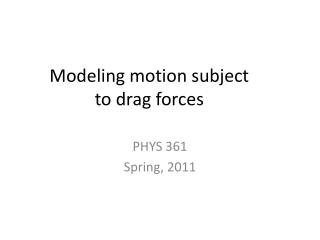Download PresentationModeling motion subject to drag forces

Loading in 2 Seconds...

# Modeling motion subject to drag forces - PowerPoint PPT Presentation

Modeling motion subject to drag forces. PHYS 361 Spring, 2011. physics. Goal is to predict the motion of an object position vs. time ... x(t) velocity vs. time ... v(t) Several forces may be acting on this object Connect motion to forces using Newton’s lawsI am the owner, or an agent authorized to act on behalf of the owner, of the copyrighted work described.
Download Presentation## Modeling motion subject to drag forces

An Image/Link below is provided (as is) to download presentation

Download Policy: Content on the Website is provided to you AS IS for your information and personal use and may not be sold / licensed / shared on other websites without getting consent from its author.While downloading, if for some reason you are not able to download a presentation, the publisher may have deleted the file from their server.

- - - - - - - - - - - - - - - - - - - - - - - - - - E N D - - - - - - - - - - - - - - - - - - - - - - - - - -
Presentation Transcript
1. Modeling motion subject to drag forces PHYS 361 Spring, 2011

2. physics • Goal is to predict the motion of an object • position vs. time ... x(t) • velocity vs. time ... v(t) • Several forces may be acting on this object • Connect motion to forces using Newton’s laws • Obtain differential equation(s): “Equations of Motion” Solution is trivial if Fnet is constant. Most interesting forces, such as those involved in riding a bicycle, are not constant.

3. deriving a useful Equation of Motion We want a differential equation of the form But Newton’s 2nd law does not, at first glance, have this form: Of course, this equation is interesting (i.e. worthy of a computational colution) only if the force is not constant. It could be a function of time, position, or even velocity. Let’s consider a situation where force depends on velocity and time. How could we rewrite Newton’s 2nd Law in the desired form?

4. Forces that depend on velocity A cyclist’s power output is more typically constant than applied force. Power is defined as Drag force: viscous and inertial viscous drag: Stokes Law. Valid for small v. pushing air out of the way valid for larger v inertial drag:

5. Equation of motion for a cyclist Assume inertial drag is much larger than viscous drag

6. Euler method Our differential equation: remember: b2 is a constant Euler method for obtaining a finite difference equation: Substituting into our equation, we can solve for vi+1